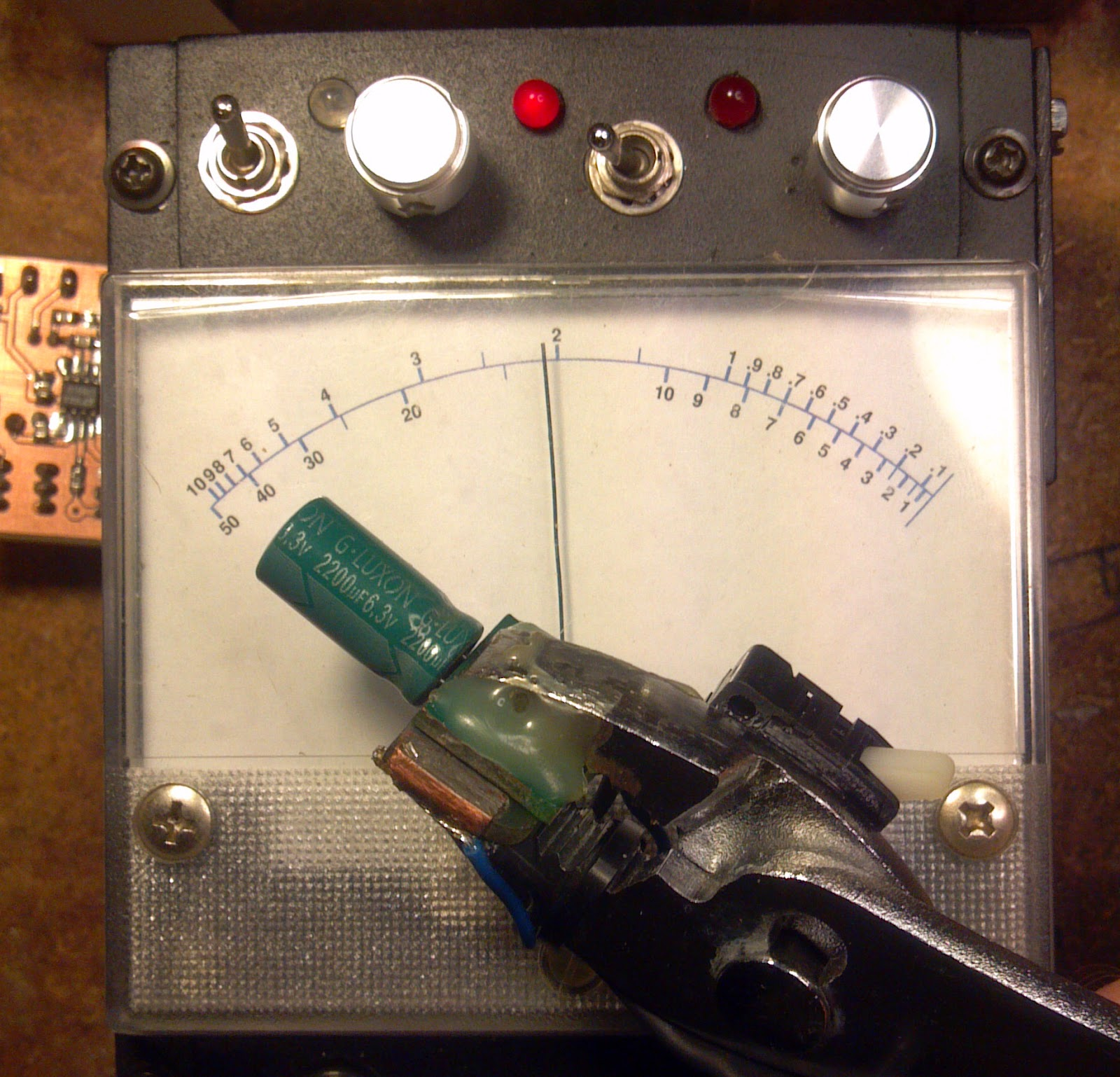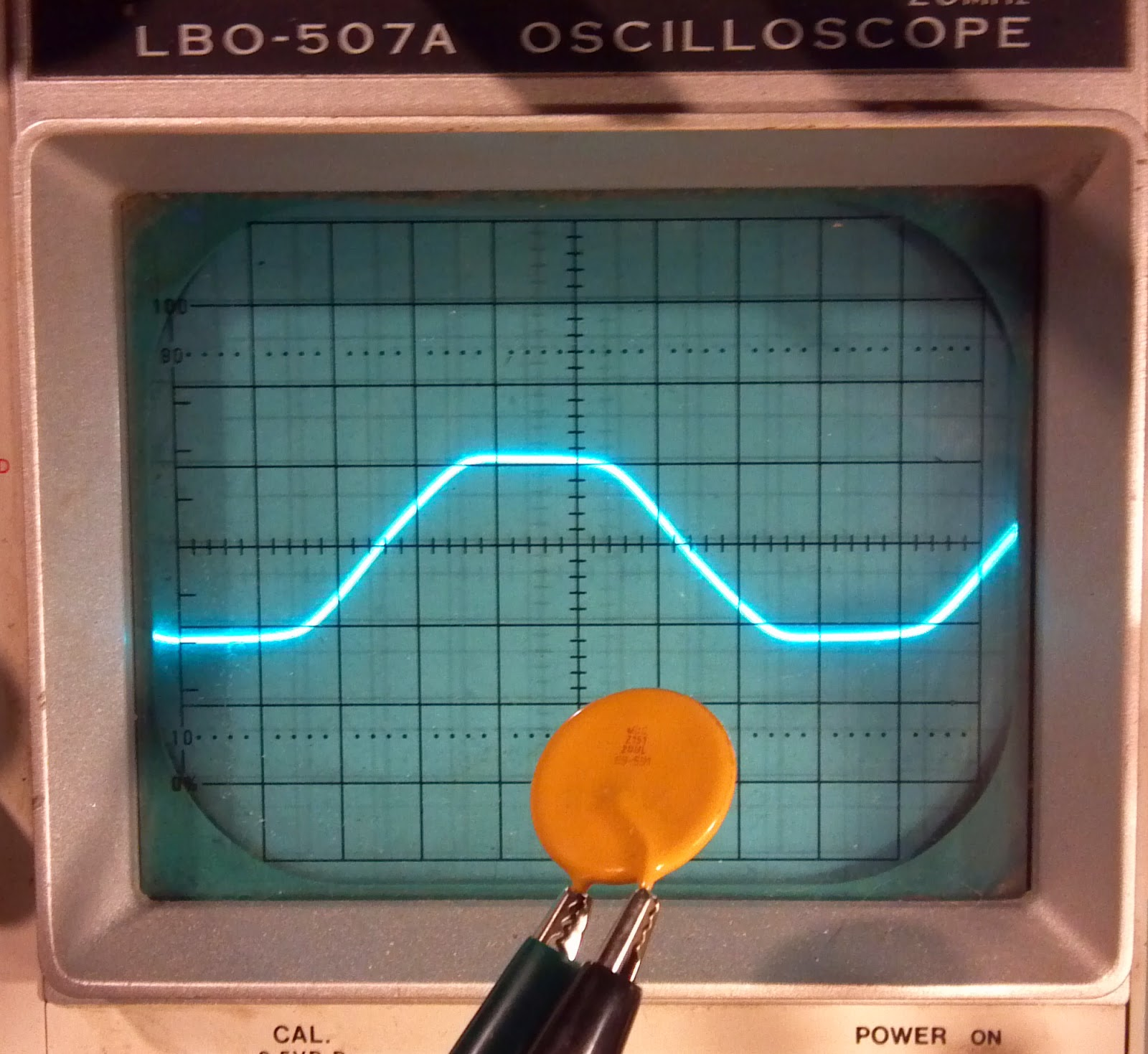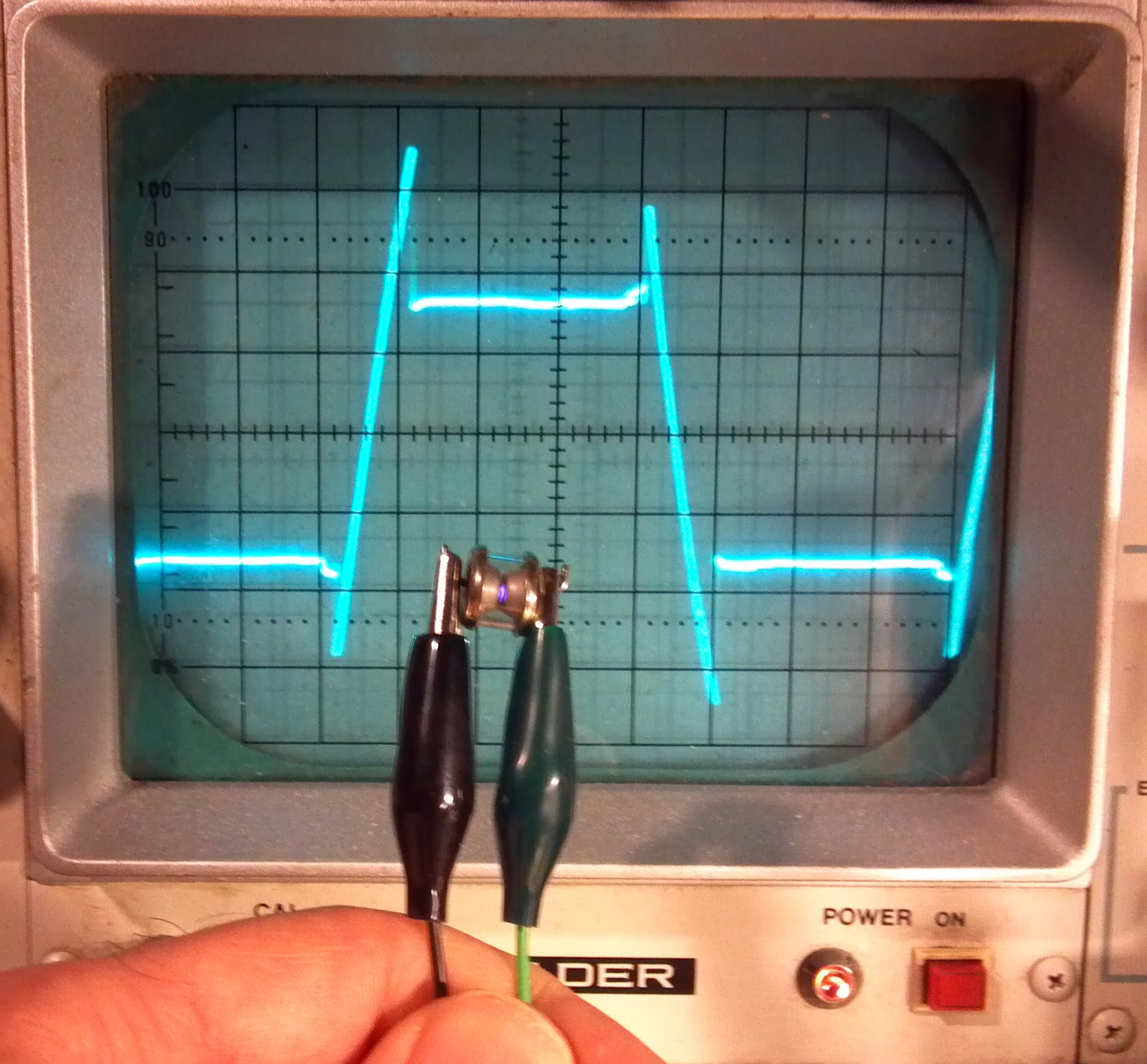## Sunday, March 29, 2015

### Create accurate custom meter legends

My ability to give a shit has worn abnormally thin lately; bear with me.  Maybe if I gain some reason to believe that this entire blog is anything more than a masturbatory distraction from an interminable hopelessness, I might bother to put some effort into things.

Analog meters are handy for making things with.  Stick it on an amplifier project or an ugly cobbled-together kludge you like to pretend is a remotely useful bit of test gear.  It doesn't matter where you use them; sometimes it's just easier to use an analog meter than a digital one.  The only real obstacle in any application is creating the corresponding legend for the meter face.  Here, I'll show my method which works well for nonlinear scales.With original meter face
In the course of finishing up my twice-aborted variant of Manfred Mornhinweg's ESR meter (I should've started from scratch), I decided that using a marker and some masking tape was just too ghetto for my tastes.  The scales in this application are not linear and I ended up needing dual scales to match the two range settings required in order to have a broad range and sufficient resolution for low resistance readings.

To start, I took a set of known resistors in the meter's range and correlated their values to the existing 0-16 linear scale.  I performed this test in both ranges, resulting in two sets of resistances and scale values.  I then measured the radius and chord length of the existing meter scale.Measure this shit so you can find section angle and legend size
Using MATLAB, the measurements are interpolated onto a new semi-logarithmic resistance space to yield a legend with convenient divisions.  Depending on the application, curve fitting, linear interpolation, or spline interpolation can be used.  I decided to just use a spline with some endslope definitions to taste.

```%% ESR meter scales
clc; clf; clear all;
format compact;

rtlo=[0.18 1 1.5 3 6.8 10 25];
rthi=[0.18 1 1.5 3 6.8 10 25 46];
xlo=[15.2 11.2 9.4 5 1 0.2 0];
xhi=[16 15.8 15.6 14.8 12.6 10 3.2 0.4];

% create semi logarithmic resistance space
%z = -1:1; d=1:9;
%R=cell2mat(arrayfun(@(i) d.*10^z(i),1:length(z),'UniformOutput',false));
R=[0 0.1 0.2 0.3 0.4 0.5 0.6 0.7 0.8 0.9 ...
1 1.5 2 2.5 3 4 5 6 7 8 9 ...
10 15 20 25 30 40 50];

% linear interpolation
%Xlo=interp1(rtlo,xlo,R);
%Xhi=interp1(rthi,xhi,R);

% or calculate from rational fit (or other function type; try cftool)
%fitlo=[-12.57 162.3 2.023 10.16];
%fithi=[-103.5 6662 -4.942e+04 -8.055 355.9 -3069];
%Xlo=(fitlo(1)*R + fitlo(2)) ./ (R.^2 + fitlo(3)*R + fitlo(4));
%Xhi=(fithi(1)*R.^2 + fithi(2)*R + fithi(3)) ./ (R.^3 + fithi(4)*R.^2 + fithi(5)*R + fithi(6));

% or use splines see if i care
Xlo=spline(rtlo,xlo,R);
Xhi=spline(rthi,[-0.15 xhi -0.10],R);

figure(1);
semilogx(rtlo,xlo,'r',R,Xlo,'c'); hold on; grid on;
semilogx(rthi,xhi,'r',R,Xhi,'c');
```Spline and original data (scale value versus resistance value)
The next step is to use plot functions to create a vector image that can be used as the final meter face. A sane person might invest this programmatic effort into directly assembling an SVG or something, but I'm just going to do it this way.
```%% curved scales
clf;
figure(2);
r=52; % scale centerline radius in mm
l=76; % scale chord length in mm
sw=2*acos(l/(2*r)); % scale swing in rad
fontsize=18;
linescale=0.5;

plot(,,'+');

axis([-40 40 0 60]);
axis equal;
for n=1:22;
if Xlo(n)<=16;
phi=sw/2 - (Xlo(n)/16)*sw + pi/2;
if sum(find(R(n)==[1.5 2.5 15 25]))==0;
offset=0.005*fontsize/10;
textstring=num2str(R(n));
if R(n) < 1;
else
end
set(t,'Rotation',(phi*180/pi)-90);
set(t,'FontWeight','bold');
set(t,'FontSize',fontsize);
end
if sum(find(R(n)==[1.5 2.5 15 25]))==0;
set(h,'LineWidth',2*linescale)
else
set(h,'LineWidth',1*linescale)
end
end
end

for n=1:length(Xhi);
if Xhi(n)<=16 && R(n)>=1;
phi=sw/2 - (Xhi(n)/16)*sw + pi/2;
if sum(find(R(n)==[1.5 2.5 15 25]))==0;
offset=0.01*fontsize/10;
set(t,'Rotation',(phi*180/pi)-90);
set(t,'FontWeight','bold');
set(t,'FontSize',fontsize);
end
if sum(find(R(n)==[1.5 2.5 15 25]))==0;
set(h,'LineWidth',2*linescale)
else
set(h,'LineWidth',1*linescale)
end
end
end

th=pi/2-sw/2:sw/100:pi/2+sw/2;
h=line(r*cos(th),r*sin(th));
set(h,'LineWidth',3*linescale)

set(h,'LineWidth',1*linescale)
```The scale as produced by the script using plot/draw tools
The font size and line weights can be set.  Number alignment can be adjusted for each scale using an offset factor.  If at this point, the results look a little off here or there, that's okay.  Once things are exported, they can be adjusted. Save the plot as a .eps file and throw it in Inkscape to adjust a few things that don't line up and then scale the output for the physical world.The scale in Inkscape after adjusting a few things
Proper print scaling should result in the X-Y scales on the original plot coming out such that they read in millimeters on the page.  A simpler way to ensure the scale size is correct is to simply print the thing and measure it to back-calculate a scaling correction factor.  Reprint the legend with the correction factor and use the original meter scales to help with positioning. Cut it to size and mount it with spray adhesive.  Turn off the lights and stop caring.Measuring a bad LUXON capacitor with the new meter face
This beats trying to eyeball things anyway.  Maybe someday I'll write about the ESR meter and the changes I made to the original design.  Maybe someday I'll have better things to do.  Maybe someday unicorns will fly out of my ass.

No wait.  Not unicorns.  That would be bad.

## Monday, March 9, 2015

### Is that a varistor or a capacitor?

It's a question that's been posed before in forums, but often the answers aren't exactly satisfying or functionally useful.  Say you have a circuit board or a new or used loose component, but you can't tell if what you're looking at is a filter capacitor or a MOV.  Sometimes they look similar, and X1 filter caps and varistors share similar locations in a circuit.  To make things worse, the part markings and appearances are often confusingly similar. I hope to try to clear up the confusion as best I can ... by hiding my contribution here where nobody will ever read it.

There are a number of things to look for first.  Contrary to common suggestion, looking for certification marks is (in my opinion) a waste of time.  They may or may not be present in either case.  A quick determination comes though if the part is marked for X1 or Y1 filter duty.  If so, it's a capacitor.

If nothing obvious comes out of the markings at first glance, let's try one quick test to make sure we're even on the right track.  Measure the resistance across the terminals.  It shouldn't be conductive.  If it is, you may be looking at a PTC or NTC thermistor or polyfuse.  You can verify this with a heat gun.Current-limiting thermistors can be identified with an ohmmeter.
Provided it's not a resistive device, look for the number code on the part.  Both capacitors and varistors may have a similar number code consisting of two significant digits and a multiplier.  For instance a code "682" expands to "6800".  If this were a capacitor, this is the capacitance in picofarads -- i.e. 6.8nF.  If this were a varistor, this would be the nominal varistor voltage at 1mA.  A 6.8 kV varistor would certainly be an uncommon find.  Some such codes would make the conclusion obvious.  Many varistors also have the voltage code prefaced by a size designation (in mm) such as 07, 10, 14, or 20.  This results in a longer code format that's uncommon in capacitor markings.Various capacitors: 1nF, 2.2nF, 4.7nF, 680pF, 4.7nF, 3.3nF.  XY mark may be on either side.
if we can assume that a part in the front end of a 120v appliance isn't likely a 6800v varistor, we might begin to recognize that there are typical ranges of values corresponding to nominal mains voltages.  A typical selection routine for a varistor on mains may be to select a part with an AC RMS withstand rating greater than 1.15 times the nominal mains voltage.  For 120v, this is a part with a 1mA rating around 220v.  For 240v, a varistor may have a 1mA rating of 470v.  Just judging by my junk drawer, 470v MOV's seem quite common.Varistors: 68v, 270v, 200v, 330v, 470v, 430v.  Markings are the 1mA voltage. Also note size markings (20mm, 14mm, 7mm)
If you're still not sure, you can check one last thing if you have a L/C meter.  Varistors act as capacitors too, but the values generally differ from what would be expected of a capacitor with the same marking.  Find the number code on the part and check to see if the device capacitance is a match.  If you have a 10mm diameter part marked "471", it should be 470pF if it's a capacitor.  If its a varistor, it will have a capacitance about half that.  Since the capacitance of a varistor depends on its diameter and thickness (obviously), don't assume it'll always be half.  Grab a random datasheet or two for a quick sanity check.

As an example, these were the measured capacitances for the pictured varistors:
• 680K :: 20mm 68v = 10nF (not a 68pF cap)
• 20K271U :: 20mm 270v = 1.65nF (not a 270pF cap)
• 14K201U :: 14mm 200v = 1.05nF (not a 200pF cap)
• G331K :: 7mm 330v = 215pF (not a 330pF cap)
• 7N431K :: 7mm 430v = 200pF (not a 430pF cap)
• 07D471K :: 7mm 470v = 110pF (not a 470pF cap)
Don't expect the measured capacitance to exactly match an offhand datasheet, but rough numbers are sufficient to indicate that none of these are capacitors.

These guidelines for marking codes are common, though not universal.  I have run across varistors where the value code denotes the working AC voltage instead of the zener voltage.  I have also run across varistors with a literal voltage value code instead of the scientific notation code.  If you really want to be sure of the part, you can put together a circuit to test the breakover characteristics of the part, but that pretty much assumes it's a MOV.  Otherwise, it will be a destructive test for sure.

As far as testing, an "octopus circuit" can be used if you have a multi-channel scope.  I only have a single scope channel, so all i use is a step-up transformer and large-value limiting resistors.  A standard F96T12 magnetic ballast can provide 370v and >490v outputs depending on configuration.  One could probably also use a diode multiplier ladder to generate a set of high test voltages.  Pay attention to the peak values and the maximum voltage allowable on the scope input and on the probes themselves.  Use sense dividers where necessary.  Don't shunt mains through the scope ground.  This method also works well for finding the breakdown voltage of gas tubesA varistor marked in nominal 150Vac (breakdown at ~240V)GTA exhibiting negative resistance characteristic
Ultimately, if you're a hobbyist and you're scrounging parts from scrapped equipment, you might be better off just pitching those questionable parts.  Consider that varistors are very inexpensive on ebay for common values, and that even a cheap chinese part is likely no more unreliable than a 20 year old used part of unknown history.  Currently 300Vac 7mm (07D471) parts can be had for \$1.90 per 10 on ebay.